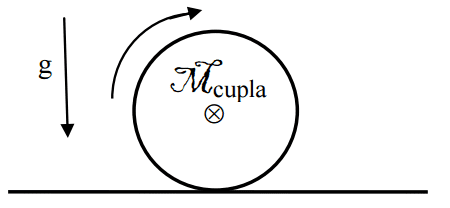# Torque and moment of inertia

• dumbdumNotSmart

## Homework Statement

Got a spicy one for you today.
There is a cylinder of mass 5kg (M) with no angular velocity and no velocity, on a surface of static friction constant μ=0.6 . It's radius is .1 meters (R). Its baricentric moment of inertia is characterized by I=.5MR^2 . Gravity is 10m/s. Kinematic μ=0.4 is also given of the flat surface.

At time t=0s, torque is applied by an external force of a magnitude of η=2 Newton meters so that it begins to roll.

Find the acceleration of the center of mass of the cylinder.
The answer in the book is a=2.67m/s^2## Homework Equations

Rototranslation
Σmoment of inertia = Iα
ma=Σforces
αR=a

## The Attempt at a Solution

Alright, so I began assuming it would begin rolling without sliding, thus we use static μ.
I sum up the inertias like so to find the angular acceleration of the cylinder.
η-μR=Iα ⇒ R(η-μR)/I=a
This gives me a negative number. I am confused. The answer in the book is a=2.67m/s^2

Why is it that simply using inertias does not work? I hear it might be done setting a fixed point and using angular momentum, but the problem is in the Rototranslation chapter.

Edit:
I believe I managed to solve it:

Ffriction=ma α=a/r
Mcupla-Ff*r=mr^2*α/2 ⇒ Mc-mar=mr*a/2 ⇒ Mc= 3mar/2 ⇒ a=2Mc/(3mr) ⇒ a=2.67m/s^2

Last edited: